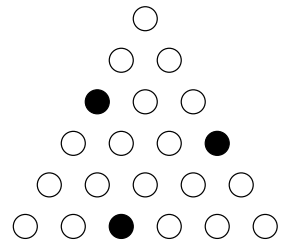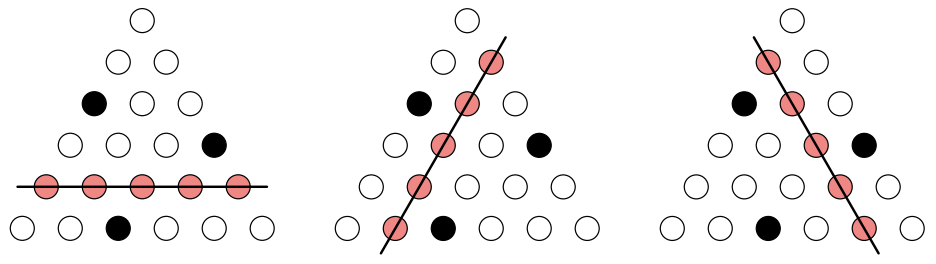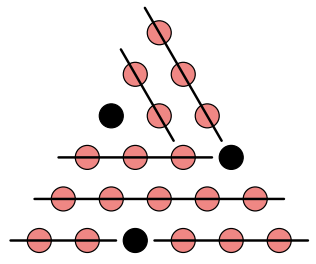시간 제한 메모리 제한 제출 정답 맞은 사람 정답 비율
8 초 (추가 시간 없음) 512 MB 0 0 0 0.000%

## 문제

You have figures that consist of white circles (◦) and black circles (•) locating in the shape of the regular triangle. An example of such figures is as follows.Figure 4: An example triangle

Now let us consider painting all the white circles into red. You can paint white circles in one step, if the white circles are on a line in parallel to one of the edges of the triangle. In order words, you can paint either from left to right, from upper-right to lower-left, or upper-right to lower-left.Figure 5: Three kinds of steps

You can paint white circles more than once, but you must not paint any black circles (i.e. the line must not meet the black circles).

The problem is that how many steps do you need to paint all the white circles in the given figures. In the case of the example above we need six steps to paint all the white circles, where one of such painting is as follows.Figure 6: An example way to paint all circles in 6 steps

## 입력

Input consists of multiple test cases.

The first line of each case contains a single positive integer N (N ≤ 15) that specifies the size of triangles. The following N lines represent a figure; the i-th line contains i characters of either w or b, where w denotes a white circle and b denotes a black circle.

Input is terminated by a line that contains a single zero, which should not be processed.

## 출력

For each case, your program should print the minimum number of steps to paint all the white circles in one line.

## 예제 입력 1

6
w
ww
bww
wwwb
wwwww
wwbwww
0


## 예제 출력 1

6